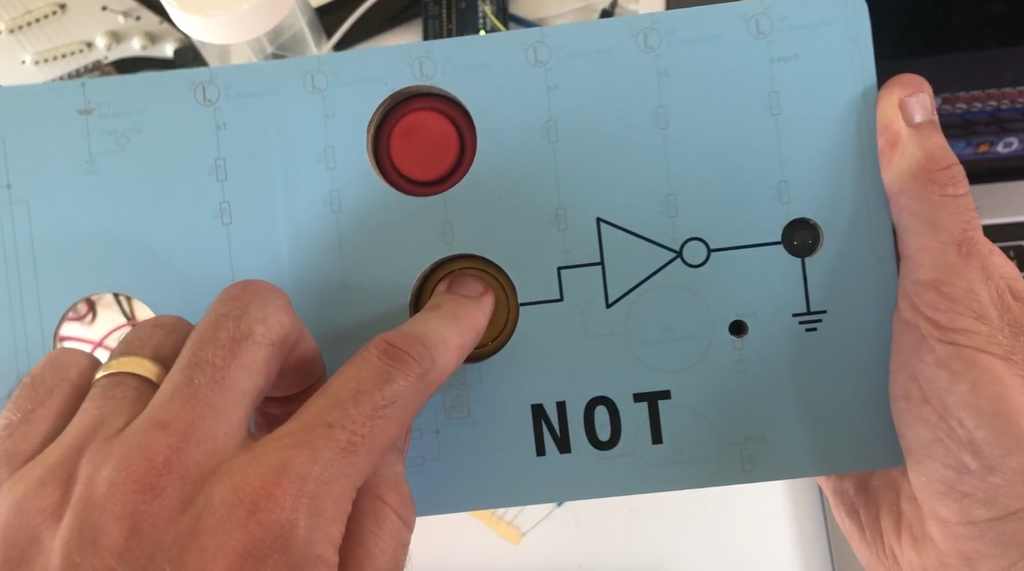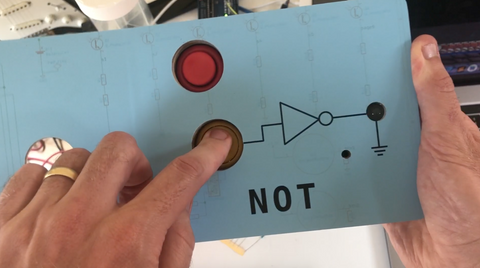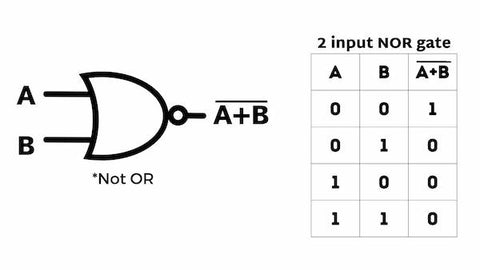# NOT Gate | Tutorial with Examples, Truth Table, and Downloadable AssetsThe NOT Gate is a digital logic gate that outputs the opposite of a single input signal.

An example of a NOT Gate would be a game of Opposites with a friend. Whatever you ask them to do, they have to do the opposite.

It would go like this:
- Input - You tell him to shut the door; Output - he opens the door.
- Input - You tell him to turn on the lights; Output - He turns off the lights.

## The NOT Gate

The NOT Gate is a digital logic gate that performs the logical negation operation on a single binary input to produce a single binary output. As the NOT Gate inverts the input signal, it is also known as Inverter

The output of a NOT Gate is HIGH (1) only when its input is LOW (0). If the input signal is HIGH (1), then the output of the NOT Gate is LOW (0).

The Boolean expression given for a NOT operation is ( – ) bar above the output, giving us the Boolean expression of Ā . So if the input signal is A, the output signal will be Ā. If A is 1, then Ā = 0; if A is 0, then Ā = 1.

Fyi, the following terms are used interchangeably
1 | HIGH | ON
O | LOW | OFF

In the truth table ON = 1 and OFF = 0. Let's show you what that looks like.

## Truth Table of the NOT Gate

The truth table of a NOT gate summarizes its behavior for all possible input combinations. The following table shows the 2-input NOT Gate truth table:## How does a NOT Gate work?

The NOT gate accomplishes this by using a transistor to switch between two states, allowing it to invert the input signal. This makes the NOT gate a fundamental building block of many digital circuits.

## Applications of NOT Gates

Here’s how we applied a NOT Gate to our book, Computer Engineering for Babies.NOT gates have several applications in digital circuits, some of which are:

• Temperature detection devices
• Decoders
• State machines
• Multiplexers
• Crystal oscillators
• CMOS inverters
• Microprocessors
• Microcontrollers
• Flip flops
• Printer IC
• Integrated circuits

## Different types of NOT Gates

NOT Gates differ based on their configurations and combinations with other gates. Some of the common NOT gates are:## Advantages of using the NOT Gate

NOT gates have several advantages in digital circuits, some of which are:

• Economical and easier to build
• Low power consumption
• Faster processing speed
• Solves complex problems quickly
• Interfaces easily compared to other logic devices

There are many ways to use a Gate in Computer Science and relating fields, here's how the NOT Gate compares to the others.

## Comparing the NOT Gate with other Logic Gates

NOT gates are one of the fundamental logic gates used in digital circuits. One of the most common ways to use NOT gates is to combine them with AND and OR gates to create universal gates (NAND and NOR gates). Universal gates can individually implement any logic circuit without using any other gate.One key difference between NOT gates and other logic gates is their output. The output of a NOT gate is always high when its input is low, and vice versa.

To the contrary, OR gates produce a high output signal when at least one input signal is high. For an AND Gate, the output is high only if all inputs are high.

Another difference is their use in Boolean algebra. NOT gates correspond to the logical negation operation in Boolean algebra. So if the input variable is 1, the result is 0, and vice-versa. OR gates correspond to addition in Boolean algebra, while AND gates correspond to Boolean multiplication.

Here’s a post we made that compares the AND OR NOT Gates in more detail.

## Final thoughts on NOT Gates

In summary, NOT gates are unique because they correspond to negation or inversion in Boolean algebra and have only one input signal.

The NOT logic gates are often combined with other basic logic gates (AND, OR, and XOR Gates) to create more intricate integrated circuits to perform complex operations.

Want us to write a post on another topic? Contact us to let us know.